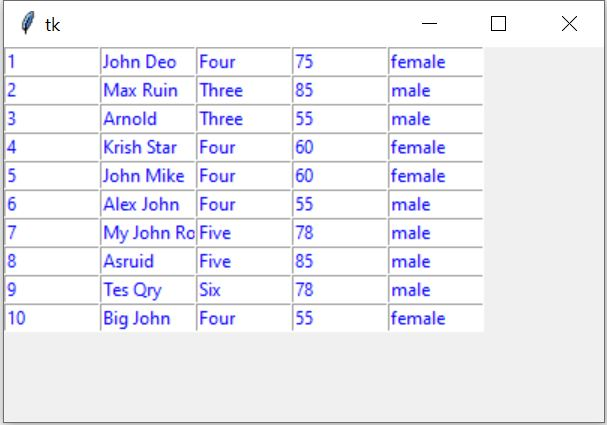# Displaying records from SQLite table

Basics of Python Tkinter

Display records from sqlite Student table in Tkinter window.## Connect to sqlite database

We connected to sqlite database and create our student table with sample data.
``````import sqlite3
my_conn = sqlite3.connect('my_db.db')``````

## using SQLAlchemy connection

``````from sqlalchemy import create_engine
#my_conn=create_engine("sqlite:////content/drive/MyDrive/db/my_db.db")
my_conn = create_engine("sqlite:///D:\\testing\\my_db\\my_db.db")``````
In windows system, the absolute path is used in above code.
The commented line is used when we keep SQLite database in Google Drive.

We will use my_conn in our further script as the connection object to get our records.

We will use Query with LIMIT to collect 10 records from the student table.

By using LIMIT Query we are restricting our number of records by getting 10 records only from SQLite database. You can change this figure to get different number of records from the database.

Note that SQLite database executes the query part only and return us one result set. This result set contains all our (10) records.
We used the returned record set i.e r_set as an iterator.

We will use one for loop to collect each row of record from the data set. Each row of data ( here student ) is a tuple. So we used another for loop to display each element of this tuple.
``````r_set=my_conn.execute('''SELECT * from student LIMIT 0,10''');
i=0 # row value inside the loop
for student in r_set:
for j in range(len(student)):
e = Entry(my_w, width=10, fg='blue')
e.grid(row=i, column=j)
e.insert(END, student[j])
i=i+1``````
This will print 10 rows of records from student table.

## Using tkinter

We will use one tkinter entry component to display each data in the window. In this variable student is a tuple and it contains one row of data. We used variable i as index for each row and variable j as each column of data.
The full code is here.
``````import sqlite3
my_conn = sqlite3.connect('my_db.db')
###### end of connection ####

##### tkinter window ######
import tkinter  as tk
from tkinter import *
my_w = tk.Tk()
my_w.geometry("400x250")

r_set=my_conn.execute('''SELECT * from student LIMIT 0,10''');
i=0 # row value inside the loop
for student in r_set:
for j in range(len(student)):
e = Entry(my_w, width=10, fg='blue')
e.grid(row=i, column=j)
e.insert(END, student[j])
i=i+1
my_w.mainloop()``````

## Using SQLAlchemy

Once the connection object my_conn is created then the code remain same in case of SQLAlchemy.
``````import tkinter  as tk
from tkinter import *
from sqlalchemy import create_engine
my_w = tk.Tk()
my_w.geometry("400x250")
my_conn = create_engine("sqlite:///D:\\testing\\my_db\\my_db.db")
r_set=my_conn.execute('''SELECT * from student LIMIT 0,10''')
i=0 # row value inside the loop
for student in r_set:
for j in range(len(student)):
e = Entry(my_w, width=10, fg='blue')
e.grid(row=i, column=j)
e.insert(END, student[j])
i=i+1
my_w.mainloop()``````

## Using Labels to display data in Tkinter window

Tkinter Label to display 10 rows of data from SQLite database student table using SELECT LIMIT query

We are using Label to display data. Here same database but from a different location we are using
``````from sqlalchemy import create_engine
from sqlalchemy.exc import SQLAlchemyError
my_path="D:\\testing\\sqlite\\my_db.db" #Change the path
my_conn = create_engine("sqlite:///" + my_path)
import tkinter  as tk
from tkinter import *
my_w = tk.Tk()
my_w.geometry("400x250")
r_set=my_conn.execute('SELECT * from student LIMIT 0,10')
i=0 # row value inside the loop
for student in r_set:
for j in range(len(student)):
e = tk.Label(my_w, width=10, fg='blue',text=student[j],anchor='w')
i=i+1
my_w.mainloop()``````

## Using MySQL or SQLite

Display ten records of student table of MySQL database on Tkinter window using SELECT & LIMIT Query

Add one Delete button to each row and onclick of the button the record will be deleted. Before deleting one message box will ask user confirmation. The record will be deleted only after user confirmation through Message box. User can cancel or click the NO button to exit the delete opration.

If we have more records to display then all the records we can’t dump or display at one go. We can break the number of records to different small sets and display the same.

Subscribe to our YouTube Channel here

## Subscribe

* indicates required
Subscribe to plus2netplus2net.com

 16-03-2021 Can you change the location of the table in the window
 23-03-2021 yes by adding starting value to i ( now it starts from 0 ) any other value , i =2. But fill with some value of row=1. Similarly you can change the value of j to 2 or 3 and fill the value of column 0 and column 1.
 16-04-2021 I don't know if im doing something wrong but this does not work for me.
 17-04-2021 What is the error you are getting ? Break this to different steps , first display the data and then add Tkinter and check where the problem is
 20-04-2021 I have no errors, just when I change the value of J and I it does not alter the position of the table.